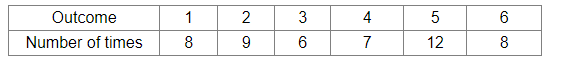# In 50 throws of a dice, the outcomes were noted as shown below:

Question:

In 50 throws of a dice, the outcomes were noted as shown below:A dice is thrown at random. What is the probability of getting an even number?

(a) $\frac{12}{25}$

(b) $\frac{3}{50}$

(C) $\frac{1}{8}$

(d) $\frac{1}{2}$

Solution:

(a) $\frac{12}{25}$

Explanation:
Total number of trials =  50
Let E be the event of getting an even number.
Then, E contains 2, 4 and 6, i.e. 3 even numbers.

$\therefore P($ getting an even number $)=P(E)=\frac{\text { Number of times even number } s \text { appear }}{\text { Total number of throws }}=\frac{(9+7+8)}{50}=\frac{24}{50}=\frac{12}{25}$